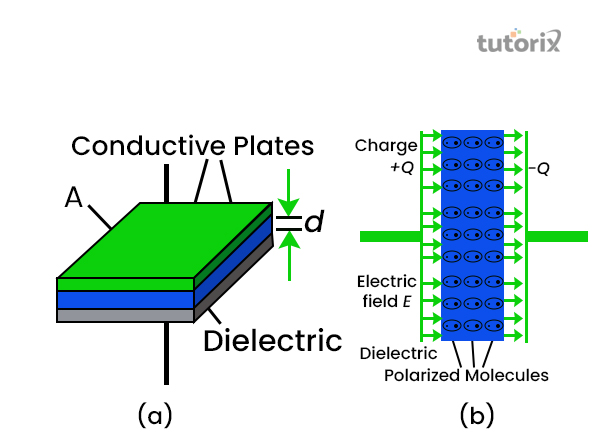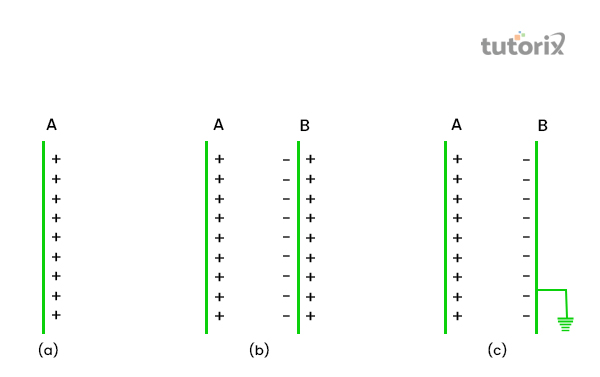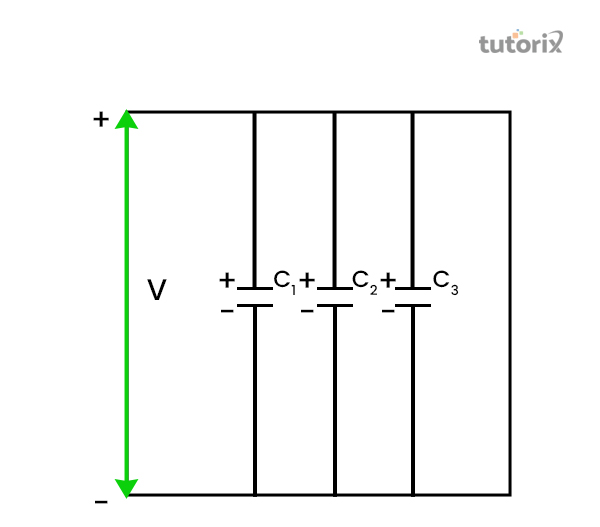# Parallel Plate Capacitor

## Introduction

A parallel plate capacitor is also called the simple form of capacitor. This kind of capacitor can be formed by using two metals or foil plates which are placed parallel to each other. The value of capacitance of the two parallel plates is fixed by the surface area of the conductive plates and the distance between the plates. Any alteration in the values causes alteration between these two particles. In this tutorial the parallel plate capacitors will be discussed further

## What are Parallel Plate Capacitors?

The parallel plate capacitors are the simple types of capacitors in existence. These capacitors have an arrangement of two electrodes which are separated by the insulating material; this material is called the dielectric medium.

The two plates in the capacitor are made of equal dimensions. These plates are connected to a power supplying unit.

According to Radivojević et al. (2018), one of the plates would be connected to a positive terminal of the battery and hence it will acquire a positive charge. The other plate is connected to a negative terminal battery and hence would acquire a negative charge (Mandrić Radivojević, 2018).

These oppositely charged plates are attracted to each other and the resultant charge is trapped within the two plates.Figure 1: Parallel Plate Capacitor

## Principle of Parallel plate capacitor

It is known that the plates can be given a certain amount of charge. If a substantially higher amount of charge is provided to the capacitor it will lead to leakage of charge. To form a parallel plate capacitor we need two plates, in case of a different plate is placed next to the positively charged plate, then the negative charge flows to the plate which is closer to the positively charged plate, and we get two charged plates, one is positively charged another is negatively charged.

Due to the presence of charge on both the plates, the negatively charged plate (plate Y) will reduce the potential difference on the positively charged plate (plate X).

Due to the presence of induction, the inner surface of plate Y becomes negatively charged and the outer surface becomes positively charged.

The negative charge of Plate Y will try to reduce the potential of Plate X, while the positive charge will try to increase the potential of plate X. When the outer surface of plate Y is earthed, the charges flow down to earth and the negative charge of the plate reduce the potential of plate X. Therefore, more amount of charge can be transferred to plate X to raise its maximum potential. This is the principle of a parallel plate capacitor.Figure 2: the principle of a parallel plate capacitor

## The formula for Parallel Plate Capacitor

The electric field direction is defined as the direction towards which the positive charge will flow (qsstudy, 2022). The capacitance of the two capacitors is associated with the limit of the plate up to which they can store the charge. As opined by Chen et al. (2019), in general, in a parallel plate capacitor, there are two metal plates with a particular surface area which is defined by A and are separated by distance d. This constitutes the basic functionality of the parallel plate capacitor. The formula is given by

$$C = k\varepsilon_{o}\frac{A}{d}$$

$\varepsilon_{o}$= ‘permittivity of the space', that is, (8.854 $×$ 10−12 F/m)

K = ‘relative permittivity of the dielectric medium of dielectric constant'

A = ‘area of the plates'

d = ‘distance between the plates'

## Multiple Parallel plate Capacitor

The arrangement of several parallel plate capacitors with a dielectric medium is placed between them for the multiple plate capacitors. The dielectric material between the conducting plates retains their functionality and prevents them from shorting out each other. Commonly, a lead is connected to the outer surface of the extreme most charged plates and the lead is connected to a voltage source, this helps in the process of charging and discharging the capacitor. Two plates can also achieve a multi-plate effect when they are rolled in a cylindrical form. The value of capacitance of multiple parallel plate capacitors is given by:

$$C=[\frac{(\varepsilon_{0}\varepsilon_{r}\: x\:A)}{D}]\times [N − 1]$$

Here, A = Area of the plates, $\epsilon_{o}$= ‘permittivity of the space' = (8.854 $×$ 10−12 F/m), $\epsilon_{r}$ = Relative Permittivity of Dielectric, D = distance between each of the plates, N = Number of platesFigure 3: Principle of parallel plate capacitor

## Dielectric material between the plates

The dipole moment of the two plates will shield the charges. Therefore, it will change the effect of the dielectric medium inserted between the plates. These materials have a certain extent of permeability which is denoted by k, which is the relative permeability.

Therefore the capacitance is denoted by

$C = \varepsilon A/d$

## Dielectric constant

The dielectric constant (K) is a particular medium given by the ratio of the permittivity of the substance, to the permittivity of the vacuum $(\epsilon_{0})$, that is $K = \epsilon/\epsilon_{0}$(electronic-tutorial, 2022). Since the permittivity of the medium and permittivity of the vacuum both have the same units (Farad/meter), the dielectric constant transforms into a dimensionless constant.

Sl No
Dielectric Material
Dielectric Constant
1Air
1
2Free space
1
3Wax paper
2
4Glass
3
5Mica
5
6Wood
7

Table 1: dielectric constant of different dielectrics

## Factors affecting the Capacitance of Parallel plate capacitor

The capacitance of a parallel plate capacitor is associated with the amount of charge the plate can retain. The greater the capacitance of the charge the greater amount of charge a capacitor can hold within itself. There it can be derived that the capacitance depends on the following factors

• The distance between the two plates which is denoted by ‘d'
• The area of the plates which is denoted by ‘A'

## Conclusion

The parallel plate capacitor is generally formed by the arrangement of two metal plates with a dielectric medium that occupies the gap between the two metal bodies and it is an insulator. This material can be air, free space or other non-conducting materials like mice, wood or paper wool. These plates are charged by a battery to establish an electric field between them forming a parallel plate capacitor.

## FAQs

Q1. When does a parallel plate capacitor act as an open circuit and short circuit?

When a parallel plate capacitor is connected to a DC source it acts as an open circuit, when it is connected to an AC source it acts as a short circuit.

Q2. What are the usages of the parallel plate capacitor?

The parallel plate capacitor can be used to filter the harmonics of the AC supply; it can be used for the tuning of the electronic circuits. This setup also acts as a source of capacitive reactive power.

Q3. What is the relation between electric charge and potential difference stored in capacitors?

The electricity charge that is stored in one of the plates of a parallel plate capacitor is directly proportional to the potential difference between the two plates present in the parallel plate capacitor.

Q4. What is the unit of Capacitance?

Capacitance is measured by the unit of Farad (F). It is named after English physicist and chemist, Michael Faraday.

## References

### Journals

Chen, X., Zhang, Z., Yu, S., & Zsurzsan, T. G. (2019, November). Fringing effect analysis of parallel plate capacitors for capacitive power transfer application. In2019 IEEE 4th International Future Energy Electronics Conference (IFEEC)(pp. 1-5). IEEE. Retrieved from: https://www.researchgate.net/profile/Tiberiu-Gabriel-Zsuzsan-2/publication/337103199_Fringing_Effect_Analysis_of_Parallel_Plate_Capacitors_for_Capacitive_Power_Transfer_Application/links/5efded5c299bf18816fa7038/Fringing-Effect-Analysis-of-Parallel-Plate-Capacitors-for-Capacitive-Power-Transfer-Application.pdf

Mandrić Radivojević, V., Rupčić, S., Srnović, M., & Benšić, G. (2018). Measuring the dielectric constant of paper using a parallel plate capacitor.International journal of electrical and computer engineering systems,9(1), 1-10. Retrieved from: https://hrcak.srce.hr/file/312732

Mandrić Radivojević, V., Rupčić, S., Srnović, M., & Benšić, G. (2018). Measuring the dielectric constant of paper using a parallel plate capacitor.International journal of electrical and computer engineering systems,9(1), 1-10. Retrieved from: https://hrcak.srce.hr/file/312732

### Websites